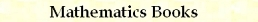Relational and Sign C/C++ Macros
by Stanislav Sýkora, Extra Byte, Via R.Sanzio 22C, Castano Primo, Italy 20022
in Stan's Library, Ed.S.Sykora, Vol.I. First release April 15, 2004
Permalink via DOI:  10.3247/SL1Soft06.002
 PREVIOUS | NEXT | Code snippets | Software Books and Links Programming Section of Stan' LIBRARY | Stan's HUB
 This document is a part of a larger collection of macros. For introductory remarks, see the first part (General Purpose Macros). For a review of macro-syntax rules and related tips and tricks, see Writing Macros.
 CATEGORY MACRO BASIC RELATIONS mIsLT, mIsLE, mIsGT, mIsGE mCompare SORTING mSort, mSort2, mSort3, mSort4, mSort5, mSort6 COMPARISONS WITH ZERO mIsNegative, mIsNonPositive, mIsPositive, mIsNonNegative SIGN FUNCTIONS mSign, mSignsAgree, mSignsDiffer mAbs, mMinusAbs, mMultiplyBySign, mAttachSign
 Basic Relations Code: #define mIsEQ(a,b) ((a)==(b)) #define mIsLT(a,b) ((a)<(b)) #define mIsLE(a,b) ((a)<=(b)) #define mIsGT(a,b) ((a)>(b)) #define mIsGE(a,b) ((a)>=(b)) #define mCompare(a,b) (mIsEQ(a,b) ? 0 : (mIsLT(a,b) ? -1 : 1)) Arguments: a and b must be of a type which is compatible with relational operators. These macros provide enhanced-readability for basic comparisons between objects of any type for which the relational operators have been defined. mIsLT(a,b), mIsLE(a,b), mIsGT(a,b), mIsGE(a,b), return the bool type true (1) or false (0). They are equivalent, respectively, to the expressions (ab), (a≥b). mCompare(a,b) provides a three-way comparison between objects. The return value is -1 when ab and 0 otherwise (note that there is no need to define the equal operator).
 Sorting Code: #define mSort(lvA,lvB,lvAux) {if (mIsLT(lvB,lvA)) mSwap(lvA,lvB,lvAux);} #define mSort2(lvA,lvB,lvAux) mSort(lvA,lvB,lvAux) #define mSort3(lvA,lvB,lvC,lvAux) \   {mSort(lvB,lvC,lvAux);mSort(lvA,lvB,lvAux);mSort(lvB,lvC,lvAux);} #define mSort4(lvA,lvB,lvC,lvD,lvAux) \   {mSort3(lvA,lvB,lvC,lvAux); \    if (mIsLT(lvD,lvA)) mRotate4(lvA,lvB,lvC,lvD,lvAux); \    else if (mIsLT(lvD,lvB)) mRotate3(lvB,lvC,lvD,lvAux); \    else if (mIsLT(lvD,lvC)) mRotate2(lvC,lvD,lvAux);} #define mSort5(lvA,lvB,lvC,lvD,lvE,lvAux) \   {mSort4(lvA,lvB,lvC,lvD,lvAux); \    if (mIsLT(lvE,lvA)) mRotate5(lvA,lvB,lvC,lvD,lvE,lvAux); \    else if (mIsLT(lvE,lvB)) mRotate4(lvB,lvC,lvD,lvE,lvAux); \    else if (mIsLT(lvE,lvC)) mRotate3(lvC,lvD,lvE,lvAux); \    else if (mIsLT(lvE,lvD)) mRotate2(lvD,lvE,lvAux);} #define mSort6(lvA,lvB,lvC,lvD,lvE,lvF,lvAux) \   {mSort5(lvA,lvB,lvC,lvD,lvE,lvAux); \    if (mIsLT(lvF,lvA)) mRotate6(lvA,lvB,lvC,lvD,lvE,LvF,lvAux); \    else if (mIsLT(lvF,lvB)) mRotate5(lvB,lvC,lvD,lvE,lvFlvAux); \    else if (mIsLT(lvF,lvC)) mRotate4(lvC,lvD,lvE,lvF,lvAux); \    else if (mIsLT(lvF,lvD)) mRotate3(lvD,lvE,lvF,lvAux); \    else if (mIsLT(lvF,lvE)) mRotate2(lvE,lvF,lvAux);} Arguments: lvA through lvF are the objects to be sorted. lvAux is an auxiliary object. Ideally, all the arguments (including lvAux) should be of the same type. They must be assignable, lvalue entities which admit the relational operator < The return values of these macros (as well as the exit values of lvAux) are dynamically dependent on the contents of the sorted entities. Consequently, the macros should not be used as constituent parts of expressions. mSort(lvA,lvB,lvAux), mSort2(lvA,lvB,lvAux), mSort3(lvA,lvB,lvC,lvAux), mSort4(lvA,lvB,lvC,lvD,lvAux), mSort5(lvA,lvB,lvC,lvD,lvE,lvAux), mSort6(lvA,lvB,lvC,lvD,lvE,lvF,lvAux), make sure that the arguments (with the exception of lvAux) are sorted to form a non-decreasing left-to-right sequence. The macros mSort and mSort2 are synonyms. Note: Should you wish to sort a larger number of items, it is likely that they would be members of an array and the task could be done better by means of an array-sorting function. The macros mSort5 and mSort6, though optimized, need not be always the best solution.
 Comparisons with zero Code: #define mIsNegative(s)    (mIsLT(s,0)) #define mIsNonPositive(s) (mIsLE(s,0)) #define mIsPositive(s)    (mIsGT(s,0)) #define mIsNonNegative(s) (mIsGE(s,0)) Arguments: s is any argument of a type for which relational comparisons to zero are defined and make sense. These macros provide enhanced-readability shorthand for sign-related properties. mIsNegative(s), mIsNonPositive(s), mIsPositive(s), mIsNonNegative(s) return the bool type true (1) or false (0).
 Sign Functions Code: #define mSign(x) (mIsNegative(x)?-1:1) #define mSignsAgree(x,y) (mIsNegative(x)? (mIsNegative(y)?1:0):(mIsNegative(y)?0:1)) #define mSignsDiffer(x,y) (mIsNegative(x)? (mIsNegative(y)?0:1):(mIsNegative(y)?1:0)) #define mAbs(x) (mIsNegative(x)?-(x):(x)) #define mMinusAbs(x) (mIsNegative(x)?(x):-(x)) #define mMultiplyBySign(x,s) (mIsNegative(s)?-(x):(x)) #define mAttachSign(x,s) (mIsNegative(s)?mMinusAbs(x):mAbs(x)) Arguments: x, y and s are arguments of any types for which comparisons with zero are defined and make sense. mSign(x) returns -1 when x<0, and +1 otherwise. For a three-way sign test (-1,0,+1), use mCompare(x,0). mSignsAgree(x,y) returns 1 when x and y have the same signs and 0 otherwise. Uses only comparisons of the type x<0 (zero is considered positive). mSignsDiffer(x,y) returns 0 when x and y have different signs and 0 otherwise. Uses only comparisons of the type x<0 (zero is considered positive). mAbs(x) returns the absolute value |x| of the argument. It uses the relational operator < between the argument x and zero, as well as the unary minus operator. These operators must be defined for the type of object x. mMinusAbs(x) returns the absolute value |x| of the argument. returns efficiently computed -mAbs(x) = -|x|. mMultiplyBySign(x,s) returns x when s is non-negative and -x otherwise. Wherever applicable, this is equivalent to the arithmetic expression sign(s)*x. mAttachSign(x,s) returns mAbs(x) when s is non-negative and mMinusAbs(x) otherwise. Wherever applicable, this is equivalent to the arithmetic expression sign(s)*abs(x).TOP | PREVIOUS | NEXT | Code snippets | Software Books and Links Programming Section of Stan' LIBRARY | Stan's HUB
 Copyright ©2004,2006 Stanislav Sýkora    DOI: 10.3247/SL1Soft06.002 Designed by Stan Sýkora